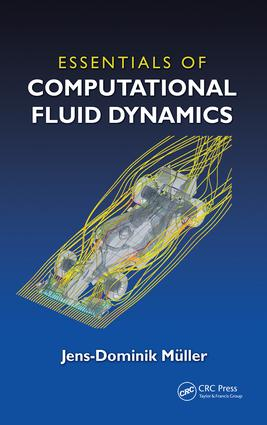# Essentials of Computational Fluid Dynamics

## 1st Edition

CRC Press

225 pages | 103 B/W Illus.

##### Purchasing Options:\$ = USD
Paperback: 9781482227307
pub: 2015-11-06
SAVE ~\$22.00
\$110.00
\$88.00
x
eBook (VitalSource) : 9780429188688
pub: 2015-11-04
from \$52.50

FREE Standard Shipping!

### Description

Covered from the vantage point of a user of a commercial flow package, Essentials of Computational Fluid Dynamics provides the information needed to competently operate a commercial flow solver. This book provides a physical description of fluid flow, outlines the strengths and weaknesses of computational fluid dynamics (CFD), presents the basics of the discretization of the equations, focuses on the understanding of how the flow physics interact with a typical finite-volume discretization, and highlights the approximate nature of CFD. It emphasizes how the physical concepts (mass conservation or momentum balance) are reflected in the CFD solutions while minimizing the required mathematical/numerical background. In addition, it uses cases studies in mechanical/aero and biomedical engineering, includes MATLAB and spreadsheet examples, codes and exercise questions. The book also provides practical demonstrations on core principles and key behaviors and incorporates a wide range of colorful examples of CFD simulations in various fields of engineering.

In addition, this author:

• Introduces basic discretizations, the linear advection equation, and forward, backward and central differences
• Proposes a prototype discretization (first-order upwind) implemented in a spreadsheet/MATLAB example that highlights the diffusive character
• Looks at consistency, truncation error, and order of accuracy
• Analyzes the truncation error of the forward, backward, central differences using simple Taylor analysis
• Demonstrates how the of upwinding produces Artificial Viscosity (AV) and its importance for stability
• Explains how to select boundary conditions based on physical considerations
• Illustrates these concepts in a number of carefully discussed case studies

Essentials of Computational Fluid Dynamics provides a solid introduction to the basic principles of practical CFD and serves as a resource for students in mechanical or aerospace engineering taking a first CFD course as well as practicing professionals needing a brief, accessible introduction to CFD.

### Reviews

"The book strikes a good balance between practical advice and mathematically oriented explanations. It covers some of the most important real-life CFD topics in depth, such as the issue of turbulence modeling, meshing, or the choice of the boundary conditions."

—Marek Behr, RWTH Aachen University, Germany

"… well written and easy to understand. It describes the basic concepts of accuracy, artificial viscosity and stability in a systematic and logical way. Moreover, the introduction of [artificial] viscosity and flux limiters are rarely found or discussed in the other CFD textbooks. …this is an excellent textbook to have for students, lecturers and practicing professionals alike. …I would like to have this book on my shelf."

—Dr. K. Djidjeli, University of Southampton, UK

"This relatively short book is intended for the user of commercial computational fluid dynamics (CFD) packages, as opposed to the developer of such programs. Müeller (Queen Mary Univ. of London, UK) taught CFD to undergraduate students for many years, and he developed the book to accompany a first course on the topic for aerospace or mechanical engineering students. The first eight chapters emphasize the basic physics and the microscopic description of the mathematical equations of fluid mechanics (both laminar and turbulent) and the description and application of finite element mesh modeling of these equations in the vicinity of various geometrical bodies, with appropriate boundary conditions. The sources of errors and the pros and cons of the various turbulent models are also described. Chapter 9 presents several case studies, and chapter 10, the appendix, is a program for a 2-D finite volume application. A short list of exercises follows each chapter. Students will need a solid grounding in basic fluid mechanics and numerical analysis to follow this text."

—CHOICE, July 2016 Issue

Introduction

CFD, the virtual windtunnel

Examples of CFD applications

Prerequisites

Literature

Ingredients

Organisation of the chapters

Exercises

Governing Equations

The physical model

Momentum equations

Simplified model equations

Exercises

Discretisation

Discretisation of the linear advection equation

Burgers' equation

Heat equation in 1-D

Advection equation in 2D

Solving the Navier-Stokes equations

The main steps in the finite volume method

Exercises

Analysis of Discretisations

Forward, Backward and Central Differences

Taylor analysis: consistency, first- and second-order accuracy

Stability and artificial viscosity, and second-order accuracy

Summary of spatial discretisation approaches

Convergence of the time-stepping iterations

Exercises

Boundary Conditions and Flow Physics

Selection of boundary conditions: a simple example

Characterisation of PDEs

Choice of boundary conditions

Exercises

Turbulence Modelling

The challenges of turbulent flow for CFD

Description of Turbulent Flow

Self-similar profiles through scaling

Velocity profiles of turbulent boundary layers

Levels of turbulence modelling

Eddy viscosity models

Near-wall mesh requirements

Exercises

Mesh Quality and Grid Generation

Influence of mesh quality on the accuracy

Requirements for the ideal mesh generator

Structured Grids

Unstructured Grids

Exercises

Analysis of the Results

Types of errors

Mesh convergence

Validation

Summary

Exercises

Case Studies

Aerofoil in 2-D, inviscid flow

Blood vessel bifurcation in 2-D

Aerofoil in 2-D, viscous flow

Appendix

Finite-volume implementation of 2-D advection

Bibliography

### About the Author

Dr. Jens-Dominik Mueller is a senior lecturer in the School of Engineering and Materials Science at Queen Mary, University of London, UK. He graduated with a Dipl.-Ing in mechanical engineering in 1989 from the Technical University of Munich, obtained a VKI Diploma in aeronautics from the Von Karman Institute in Brussels in 1990, and an MSc and PhD in aerospace engineering from the University of Michigan, Ann Arbor, in 1996. He held research and academic positions at CERFACS, Toulouse, Oxford University and Queen's University Belfast. He is the author of more than 40 publications and has organized numerous international conferences.

### Subject Categories

##### BISAC Subject Codes/Headings:
TEC009070
TECHNOLOGY & ENGINEERING / Mechanical